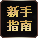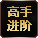[an error occurred while processing the directive] [an error occurred while processing the directive]
 快速通道 专区首页 数 据 库 文章投稿 账号注册 截图投稿 相关下载
 游戏资料 怪物图鉴 副本攻略 职业介绍 技能说明 游戏系统 游戏地图 游戏任务 BOSS图鉴 公会详解 生活技能 装备系统 装备图鉴
 玩家互动 游戏专题 文章导航 经验心情 职业文章 精华文章 火爆论坛
 截图视频 视频专区 游戏截图 原画壁纸# 3.0精灵BB技能伤害揭秘 职业伤害公式

3.0版本，60等级火宝宝2700增幅，各命令伤害如下：

A)雷电爪V技能(5)说明伤害(17x5+783)=868x1.15=998，实际伤害2994 (雷电爪，智力以135计算)，0.6秒吟唱，12秒CD。

3.0版本，60等级火宝宝2700增幅，各命令伤害如下：

A)雷电爪V技能(5)说明伤害(17x5+783)=868x1.15=998，实际伤害2994 (雷电爪，智力以135计算)，0.6秒吟唱，12秒CD。

B)扰乱V技能(1)说明伤害(24x1+1157)=1181x1.15=1358，实际伤害4988(扰乱，智力以101计算)，0.8秒吟唱，18秒CD。

C)爆裂爪V技能(1)说明伤害(34x1+1628)=1204x1.15=1662，实际伤害6105(爆裂爪，智力以101计算)，1.5秒吟唱，24秒CD。

A)雷电爪：2994/3.25=921伤害，0.6秒吟唱，12秒CD

B)扰乱：4988/3.25=1535，0.8秒吟唱，18秒CD

C)爆裂爪：6105/3.25=1878，1.5秒吟唱，24秒CD

A)雷电爪0.6x60=36秒吟唱，12x60=720秒CD，伤害2994x60=179640

B)扰乱0.8x40=32秒吟唱，18x40=720秒CD，伤害4988x40=199520

C)爆裂爪1.5x30=45秒吟唱，24x30=720秒CD，伤害6105x30=183150

60等级角色，2700增幅地狱火II技能(7)说明伤害(56x7+2507)=2899x1.2=3479，实际伤害3479x3.25=11307

1)地狱火II技能(7)说明伤害(56x7+2507)=2899x1.2=3479，实际伤害11307

pve：技能伤害 x (1+<增幅/智力x10>) x (1-对方魔法防御) =实际伤害

pvp：技能伤害 x (1+<增幅/智力x10>) x (1-对方魔法防御) x (对方PC-我方PC) x(pvp惩罚系数0.7) x(技能本身的pvp惩罚系数- 某些技能有，某些技能没有) =实际伤害

【永恒之塔专区首页】最新消息第一时间推送给你